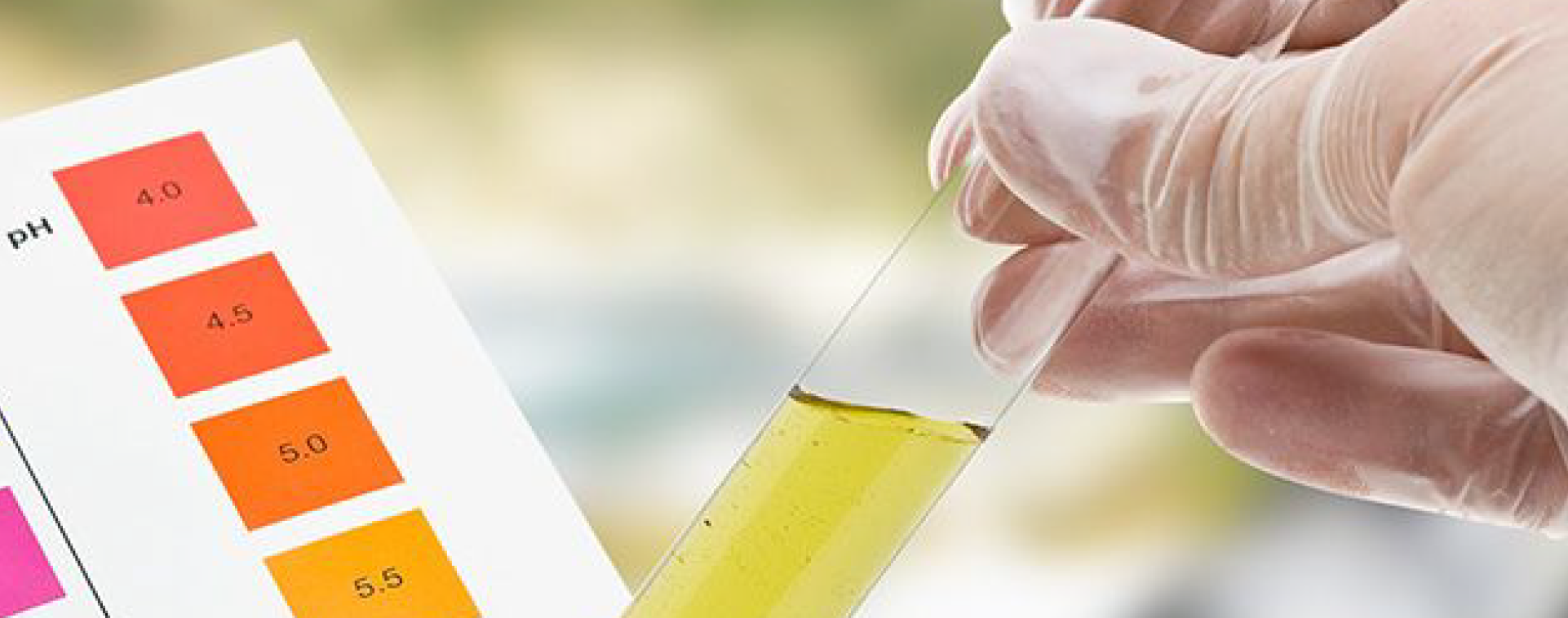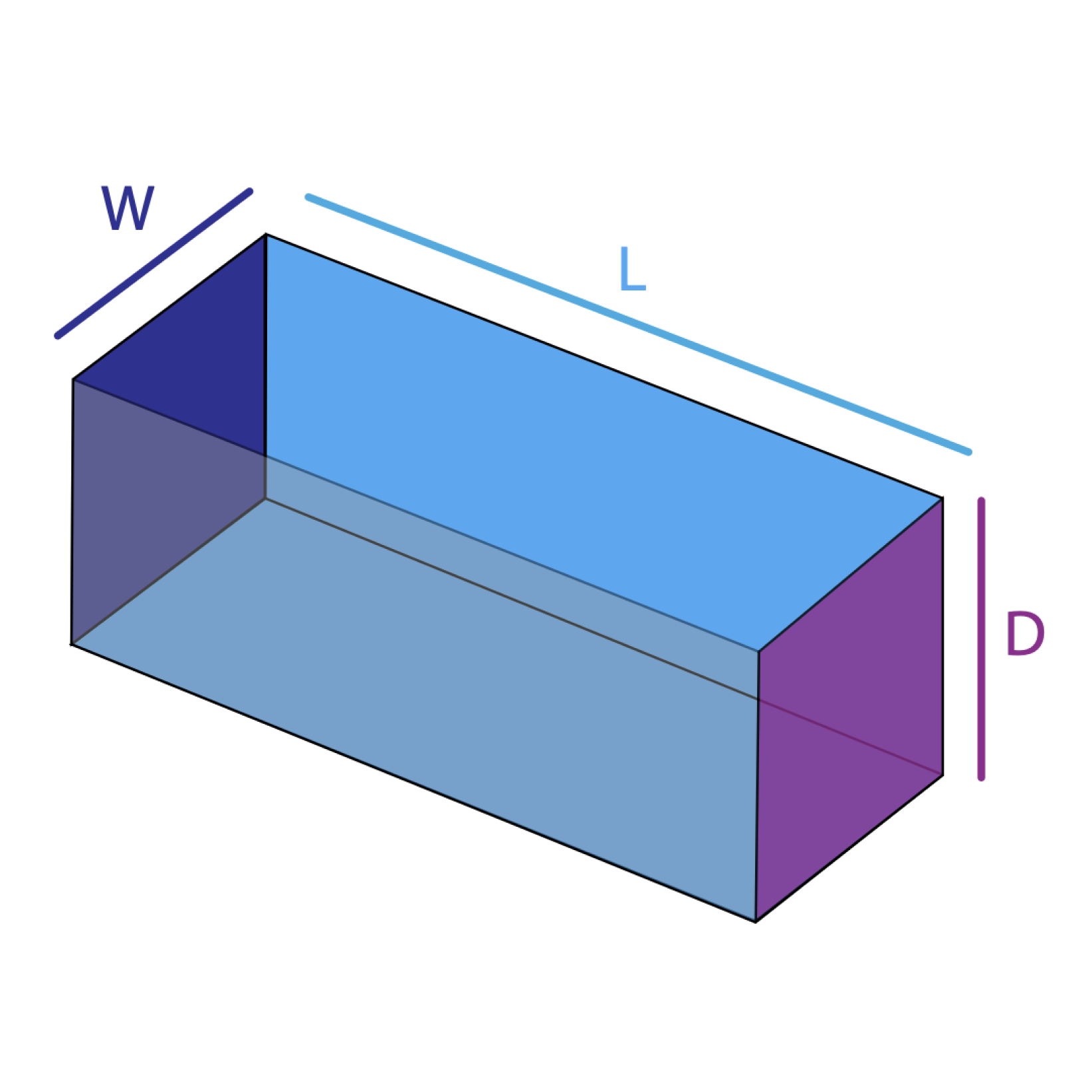# Pond size calculator

Pond Size (ft):
Pond Length ______ft
x Pond Width ______ft
× Pond Depth ______ft
× 6.25 = ______Gallons
× 4.54 = ______Litres
Pond Size (m):
Pond Length ______m
x Pond Width ______m
× Pond Depth ______m
× 220 = ______Gallons
× 4.54 = ______Litres

When calculating the size of your pond use the average length, width and depth.